# Criticality Analysis

## Contents

### Introduction

Failure mode criticality, as used in FMECA, can be generically described as the joint probability of three events:

1. The probability of failure of the component.
2. The probability that the mode under consideration was the culprit.
3. The probability that this mode would result in a system failure (which for the case of non-redundancy or if in series =1).

This number is then used to provide relative rankings for the different failure modes. This general approach is described in Kececioglu and expanded further here.

### General Criticality Number Computation

Mathematically, and for a specific failure mode,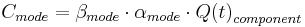${{C}_{mode}}={{\beta }_{mode}}\cdot {{\alpha }_{mode}}\cdot Q{{(t)}_{component}} \,\!$

where:

•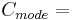${{C}_{mode}}= \,\!$ Criticality number for the failure mode.
•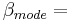${{\beta }_{mode}}= \,\!$ Conditional probability of mission loss.
•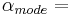${{\alpha }_{mode}}= \,\!$ Failure mode ratio.
•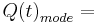$Q{{(t)}_{mode}}= \,\!$ Unreliability or probability of failure due to that mode at time t.
•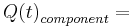$Q{{(t)}_{component}}= \,\!$ Unreliability or probability of failure of the component at time t.

In the case that the probability of occurrence of each mode is defined, and the modes are assumed to be in a series, then a better way to obtain a criticality value is: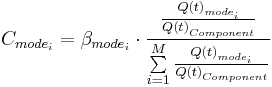${{C}_{{{mode}_{i}}}}={{\beta }_{{{mode}_{i}}}}\cdot \frac{\frac{Q{{(t)}_{{{mode}_{i}}}}}{Q{{(t)}_{Component}}}}{\sum\limits_{i=1}^{M}{\frac{Q{{(t)}_{{{mode}_{i}}}}}{Q{{(t)}_{Component}}}}}\,\!$

Note that this differs from the specialized definition given in Task 102 of MIL-STD-1629A, Section 3.2.1.6 (described next).

Specifically, a procedure is given for computing the “Failure mode criticality number” when an assumption of a constant failure mode (i.e., an exponential distribution) is used and for small values of lambda:

This is given by: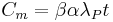${{C}_{m}}=\beta \alpha {{\lambda }_{P}}t\,\!$

where:

•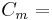${{C}_{m}}=\,\!$ Criticality number for a failure mode.
•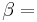$\beta =\,\!$ Conditional probability of mission loss.
•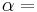$\alpha =\,\!$ Failure mode ratio.
•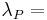${{\lambda }_{P}}=\,\!$ Part failure rate.
•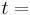$t=\,\!$ Mission Duration.

In addition to the constant failure rate assumption, it is important to note that this formulation assumes that the part failure rate values for a mode are relatively small and thus the following simplification is made:$Q(t)=1-{{e}^{-{{\lambda }_{P}}\cdot t}}\approx {{\lambda }_{P}}\cdot t \,\!$

This approximation holds true when using an exponential distribution and small values of lambda (<1E-4), but not for all values. ReliaSoft chose to use the general formulation instead of the specialized and limited 1629A formulation.

### Example

To illustrate this assume that a component has three failure modes (FM1, FM2 and FM3) where any mode can cause system failure (i.e., a series system). The unreliability is defined by the failure model of each mode at t=1,000 hours. (In this case FM1 is Weibull ($\beta=3\,\!$,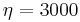$\eta=3000\,\!$ hrs) and FM2 and 3 are exponential with MTTF=10,000 hours.)

Then: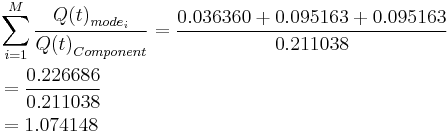\begin{align} & \sum\limits_{i=1}^{M}{\frac{Q{{(t)}_{{{mode}_{i}}}}}{Q{{(t)}_{Component}}}}=\frac{0.036360+0.095163+0.095163}{0.211038} \\ & =\frac{0.226686}{0.211038} \\ & =1.074148 \end{align}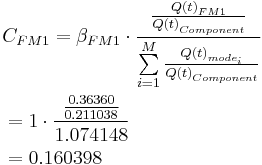\begin{align} & {{C}_{FM1}}={{\beta }_{FM1}}\cdot \frac{\frac{Q{{(t)}_{FM1}}}{Q{{(t)}_{Component}}}}{\sum\limits_{i=1}^{M}{\frac{Q{{(t)}_{{{mode}_{i}}}}}{Q{{(t)}_{Component}}}}} \\ & =1\cdot \frac{\frac{0.36360}{0.211038}}{1.074148} \\ & =0.160398 \end{align}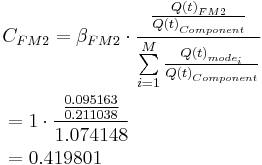\begin{align} & {{C}_{FM2}}={{\beta }_{FM2}}\cdot \frac{\frac{Q{{(t)}_{FM2}}}{Q{{(t)}_{Component}}}}{\sum\limits_{i=1}^{M}{\frac{Q{{(t)}_{{{mode}_{i}}}}}{Q{{(t)}_{Component}}}}} \\ & =1\cdot \frac{\frac{0.095163}{0.211038}}{1.074148} \\ & =0.419801 \end{align}

and: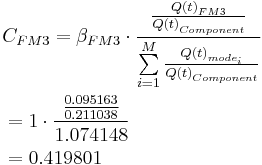\begin{align} & {{C}_{FM3}}={{\beta }_{FM3}}\cdot \frac{\frac{Q{{(t)}_{FM3}}}{Q{{(t)}_{Component}}}}{\sum\limits_{i=1}^{M}{\frac{Q{{(t)}_{{{mode}_{i}}}}}{Q{{(t)}_{Component}}}}} \\ & =1\cdot \frac{\frac{0.095163}{0.211038}}{1.074148} \\ & =0.419801 \end{align}

### Derivation

Given that the component failed before time T, the probability that it is caused by mode B is: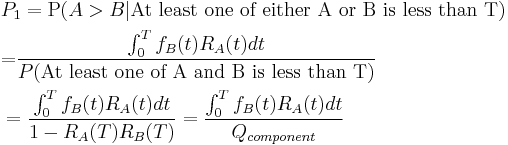\begin{align} & {{P}_{1}}=\operatorname{P}(A>B|\text{At least one of either A or B is less than T)} \\ & \text{=}\frac{\int_{0}^{T}{{{f}_{B}}(t){{R}_{A}}(t)dt}}{P(\text{At least one of A and B is less than T})} \\ & =\frac{\int_{0}^{T}{{{f}_{B}}(t){{R}_{A}}(t)dt}}{1-{{R}_{A}}(T){{R}_{B}}(T)}=\frac{\int_{0}^{T}{{{f}_{B}}(t){{R}_{A}}(t)dt}}{{{Q}_{component}}} \end{align}

The upper bound of P1 is: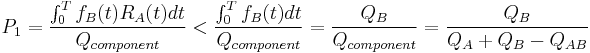${{P}_{1}}=\frac{\int_{0}^{T}{{{f}_{B}}(t){{R}_{A}}(t)dt}}{{{Q}_{component}}}<\frac{\int_{0}^{T}{{{f}_{B}}(t)dt}}{{{Q}_{component}}}=\frac{{{Q}_{B}}}{{{Q}_{component}}}=\frac{{{Q}_{B}}}{{{Q}_{A}}+{{Q}_{B}}-{{Q}_{AB}}}$.

The lower bound of P1 is: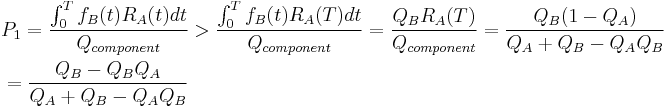\begin{align} & {{P}_{1}}=\frac{\int_{0}^{T}{{{f}_{B}}(t){{R}_{A}}(t)dt}}{{{Q}_{component}}}>\frac{\int_{0}^{T}{{{f}_{B}}(t){{R}_{A}}(T)dt}}{{{Q}_{component}}}=\frac{{{Q}_{B}}{{R}_{A}}(T)}{{{Q}_{component}}}=\frac{{{Q}_{B}}(1-{{Q}_{A}})}{{{Q}_{A}}+{{Q}_{B}}-{{Q}_{A}}{{Q}_{B}}} \\ & =\frac{{{Q}_{B}}-{{Q}_{B}}{{Q}_{A}}}{{{Q}_{A}}+{{Q}_{B}}-{{Q}_{A}}{{Q}_{B}}} \\ \end{align}

Thus, the probability that the failure is caused by mode B is:$\frac{{{Q}_{B}}-{{Q}_{B}}{{Q}_{A}}}{{{Q}_{A}}+{{Q}_{B}}-{{Q}_{A}}{{Q}_{B}}}<{{P}_{1}}<\frac{{{Q}_{B}}}{{{Q}_{A}}+{{Q}_{B}}-{{Q}_{AB}}}$

Thus the approximation: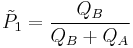${{\tilde{P}}_{1}}=\frac{{{Q}_{B}}}{{{Q}_{B}}+{{Q}_{A}}}$

is within the bounds.

Similarly, given that the component failed before time T, the probability that it is caused by mode A is: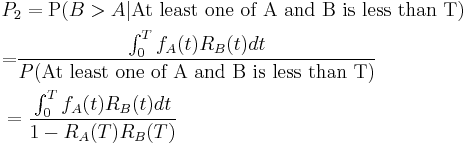\begin{align} & {{P}_{2}}=\operatorname{P}(B>A|\text{At least one of A and B is less than T)} \\ & \text{=}\frac{\int_{0}^{T}{{{f}_{A}}(t){{R}_{B}}(t)dt}}{P(\text{At least one of A and B is less than T})} \\ & =\frac{\int_{0}^{T}{{{f}_{A}}(t){{R}_{B}}(t)dt}}{1-{{R}_{A}}(T){{R}_{B}}(T)} \end{align}

Then the sum of P1 and P2 is: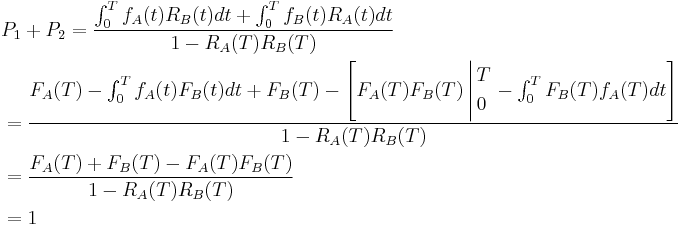\begin{align} & {{P}_{1}}+{{P}_{2}}=\frac{\int_{0}^{T}{{{f}_{A}}(t){{R}_{B}}(t)dt}+\int_{0}^{T}{{{f}_{B}}(t){{R}_{A}}(t)dt}}{1-{{R}_{A}}(T){{R}_{B}}(T)} \\ & =\frac{{{F}_{A}}(T)-\int_{0}^{T}{{{f}_{A}}(t){{F}_{B}}(t)dt}+{{F}_{B}}(T)-\left[ {{F}_{A}}(T){{F}_{B}}(T)\left| \begin{align} & T \\ & 0 \\ \end{align} \right.-\int_{0}^{T}{{{F}_{B}}(T){{f}_{A}}(T)dt} \right]}{1-{{R}_{A}}(T){{R}_{B}}(T)} \\ & =\frac{{{F}_{A}}(T)+{{F}_{B}}(T)-{{F}_{A}}(T){{F}_{B}}(T)}{1-{{R}_{A}}(T){{R}_{B}}(T)} \\ & =1 \\ \end{align}

Thus the approximation: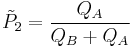${{\tilde{P}}_{2}}=\frac{{{Q}_{A}}}{{{Q}_{B}}+{{Q}_{A}}}$

is within these bounds.

Also note: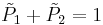${{\tilde{P}}_{1}}+{{\tilde{P}}_{2}}=1$